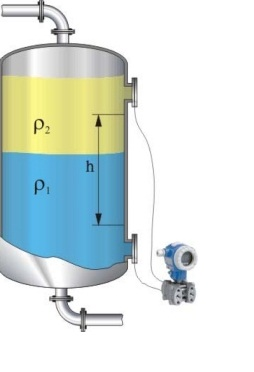# Interface Level Measurement### INTRODUCTION

Differential pressure (DP) transmitters are used to measure the interface of two fluids that have different specific gravities (S). To make an interface measurement, the overall level must be at or above the low pressure tap at all times. It is important that the level be large enough to create a reasonable DP between the two specific gravity extremes. This measurement can be done with or without remote seals. However, from a maintenance standpoint, it may be easier to use a remote seal assembly; keeping the wet leg at a constant height can be difficult in some applications.

### SITUATION

A vessel requires an interface measurement where the level is 150 inches. The vessel has two fluids with specific gravities of 1.0 and 1.08.To determine the calibrated range for the transmitter, four assumptions need to be made:
1. At the lower calibrated value, 4 mA point, the tank is filled with the lighter fluid.
2. At the upper calibrated value, 20 mA point, the tank is filled with the heavier fluid.
3. The taps leading to the transmitter are flooded at all times. The overall level should be equal to or higher than the upper (low pressure) tap.
4. There is always a reference level seen by the low pressure side. This can be accomplished with either a remote seal system or a wet leg. The reference level must have a constant height and density. The calculation is modified slightly for wet leg systems when the density of the high and low pressure wet legs differ.

The measured level is composed of a combination of the two fluids:
L = L1S1 + L2S2

•  When the tank is filled with the lighter fluid, the transmitter will be at 4 mA (or 0% of span) and L2 = L:
HP = L2S2 + dSf
LP = dSf + hSf
4 mA DP = HP – LP = L2S2 – hSf
DP = (150 x 1.0) – (150 x 0.934)
4 mA = 9.9 inH2O
•  When the tank is filled with the heavier fluid, the transmitter will be at 20 mA (or 100% of span) and           L1 = L:
HP = L1S1 + dSf
LP = dSf + hSf
20 mA DP = HP – LP = LS1 – hSf
DP = (150 x 1.08) – (150 x 0.934)
20 mA = 21.9 inH2O
The calibrated span is 9.9 to 21.9 inH20. When the transmitter reads 9.9 inH20, the tank is filled with the lighter fluid. When the transmitter reads 21.9 inH20, the tank is filled with the heavier fluid.

To determine where the interface of a mixture of fluids is, use the DP reading as a percent of span. For example, if the transmitter output is 18.4 mA or a DP of 20.7 inH2O, the interface is calculated by using the formula: